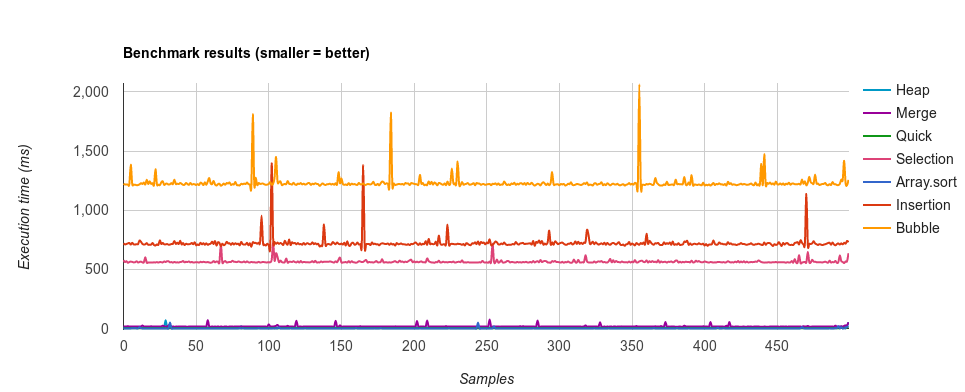{{ message }}

# eugenmihailescu / sorting-algorithms

JS sorting algorithms (Bubble | Insertion | Selection | Merge | Heap | Quick)

## Files

Failed to load latest commit information.
Type
Name
Commit time

# sorting-algorithms

Classes for sorting algorithms (bubble|insertion|quick|merge)

This repository features a lightweight JavaScript implementation of the most common sorting algorithms. For their usage considerations they extend the standard JavaScript Array prototype with the following functions:

• insertionsort (compare)
• bubblesort (compare)
• quicksort (compare)
• mergesort (compare)
• heapsort (compare)
• selectionsort (compare)
• pigeonholesort (compare)
• bucketsort (size,compare)

where the optional paramter `compare` can be either a function, a boolean or entirely omitted. When a function is provided then it is used for item comparison. When provided as a boolean `true` then the array is sorted descendently. Otherwise (default when omitted) an ascedent (the `>` operator) order is assumed. The optional `size` parameter is the number buckets where the items are scattered.

Usage example:

```var a = [6, 5, 3, 1, 8, 7, 2, 4];

// implicit ascendent sorting
a.bubblesort();

// explicit descendent sorting
a.heapsort(true);

// explicit sorting using a compare function
a.quicksort(function(m, n) {
return m > n;
});```

The algorithms time complexity, its simplicity and performance:

Algorithm Best case Worst case Simplicity Speed
Pigeonhole* n+k n+k simple *****
QuickSort n*log(n) n^2 complex *****
Bucketsort* n+k n^2 simple *****
MergeSort n*log(n) n*log(n) moderate ****
HeapSort n*log(n) n*log(n) complx ****
Insertion n n^2 simple ***
Selection n^2 n*^2 simple **
BubbleSort n-1 n(n-1)/2 simple *

where `k` is the range of key values and `n` is the input size.

(*) Pigeonhole supports only numeric values.

A benchmark demo application is included. It allows to compare the performances of the algorithms by displaying the results in a graph (via Google Chart API).

Features:

• testing aginst randomly generated arrays (string|integer)
• allows running against various array's sizes (100-100k items)
• allows running against several sample data (1-1000) to get a statically valid probability of their performance
• allows running in parallel threads by using Web Worker
• displays the results both numerically and graphically (as bar, stacked bar, lines)
• the graphs can be zoomed at various levels
• export as SVG|PNGThe demo is very usefull if you want to compare the algorithms' performances on various browsers/OS (the JavaScript engines usually differ from browser to browser).

JS sorting algorithms (Bubble | Insertion | Selection | Merge | Heap | Quick)